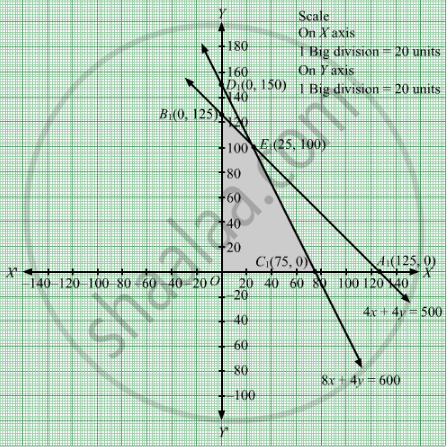# A Firm Manufactures Two Products a and B. Each Product is Processed on Two Machines M1 and M2. Product a Requires 4 Minutes of Processing Time on M1 and 8 Min. - Mathematics

Sum

A firm manufactures two products A and B. Each product is processed on two machines M1 and M2. Product A requires 4 minutes of processing time on M1 and 8 min. on M2 ; product B requires 4 minutes on M1 and 4 min. on M2. The machine M1 is available for not more than 8 hrs 20 min. while machine M2 is available for 10 hrs. during any working day. The products A and B are sold at a profit of Rs 3 and Rs 4 respectively.
Formulate the problem as a linear programming problem and find how many products of each type should be produced by the firm each day in order to get maximum profit.

#### Solution

Let x products of type A and y products of type are manufactured.
Number of products cannot be negative.
Therefore, $x, y \geq 0$

The given information can be tabulated as f

 Product $M_1$ (minutes) $M_2$ (minutes) A(x) 4 8 B(y) 4 4 Availability 500 600

Therefore, the constraints areollows:

$4x + 4y \leq 500$

$8x + 4y \leq 600$

The products A and B are sold at a profit of Rs 3 and Rs 4 respectively. Therefore, Profit gained from x products of type A and y products of type B is Rs 3x and Rs 4y respectively.
Total profit = Z = 3x + 4y which is to be maximised.

Thus, the mathematical formulat​ion of the given linear programmimg problem is

Max Z =  $3x + 4y$
subject to

$4x + 4y \leq 500$

$8x + 4y \leq 600$

First we will convert inequations into equations as follows:
4x + 4y = 500, 8x + 4y = 600, x = 0 and y = 0

Region represented by 4x + 4y ≤ 500:
The line 4x + 4y = 500 meets the coordinate axes at A1(125, 0) and B1(0, 125) respectively. By joining these points we obtain the line
4x + 4y = 500. Clearly (0,0) satisfies the 5x + 20y = 400 . So, the region which contains the origin represents the solution set of the inequation 5x + 20y ≤ 400.

Region represented by 8x + 4y ≤ 600:
The line 8x + 4y = 600 meets the coordinate axes at C1(75, 0) and D1(0, 150) respectively. By joining these points we obtain the line
8x + 4y = 600. Clearly (0,0) satisfies the inequation 8x + 4y ≤ 600. So,the region which contains the origin represents the solution set of the inequation
8x + 4y ≤ 600.

Region represented by x ≥ 0 and y ≥ 0:
Since, every point in the first quadrant satisfies these inequations. So, the first quadrant is the region represented by the inequations x ≥ 0, and ≥ 0.
The feasible region determined by the system of constraints 4x + 4y ≤ 500, 8x + 4y ≤ 600, x ≥ 0, and y ≥ 0 are as follows.The corner points are O(0, 0), B1(0, 125), E1(25, 100) and C1(75, 0).

The values of Z at these corner points are as follows

 Corner point Z= 3x + 4y O 0 B1 500 E1 475 C1 225

The maximum value of Z is 500 which is attained at B1(0, 125).
Thus, the maximum profit is Rs 500 obtained when no units of product A and 125 units of product B were manufactured.

Concept: Graphical Method of Solving Linear Programming Problems
Is there an error in this question or solution?

#### APPEARS IN

RD Sharma Class 12 Maths
Chapter 30 Linear programming
Exercise 30.4 | Q 12 | Page 52